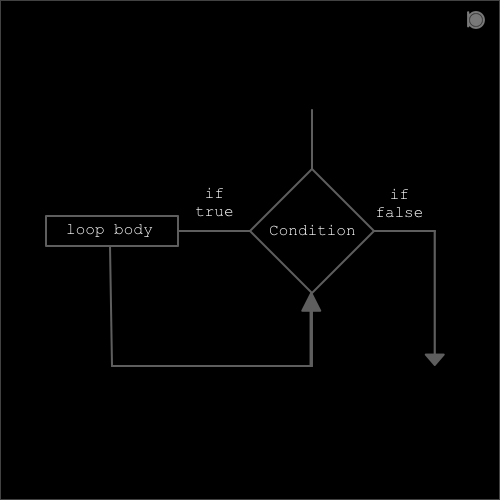while loop is used to execute blocks of statements repeatedly until the condition returns false.In the while loop the condition is checked first and if the condition is true then the loop body is executed. Also if the condition is false then the loop body is never executed.

##### Syntax for while loop in C:
``````while(condition or expression)
{
statements;
}
``````

Here condition or expression can be true or false.

### Program example of while loop

``````//An example of while loop
#include<stdio.h>

int main()
{
int a = 1;
while (a<10)
{
printf("%d\n",a);
a++;
}
return 0;
}
``````

### output

``````1
2
3
4
5
6
7
8
9
``````

In above example we have a variable `a = 1`. In the while loop we have provided a condition `a<10` which means the condition will be true till the value of `a` is less than `10`. This means if the value of `a` becomes `10` then the condition will become false and will not execute the loop body. Inside the loop, the loop body consists of two statements:

``````printf("%d\n",a);
a++;
``````

The first statement will print the value of `a`. Initially the value of a is 1 and is printed as output. After that second statement `a++` will be executed which means the value of a is post incremented by 1 and becomes 2. After the execution of last statement in the loop body the control is then transferred for the condition check.

Now if we compare the condition `a<10` we have `a = 2` so the condition is true and again the loop body is executed and the output is 2. And in this way the output is printed till the value of a is 9. Now when the value of a is 10 then our condition becomes false so the loop body is not executed and the control is transferred to the statements that occurs right after the while loop.

### For example:

``````//An example of infinite while loop
#include<stdio.h>

int main()
{
while(1)
{
printf("OjhaBikash\n");
}
return 0;
}
``````

### output

``````OjhaBikash
OjhaBikash
OjhaBikash
OjhaBikash
OjhaBikash
OjhaBikash
OjhaBikash
...
...
.
.
.
``````

`Note: To exit from an infinite loop press ctrl+c.`